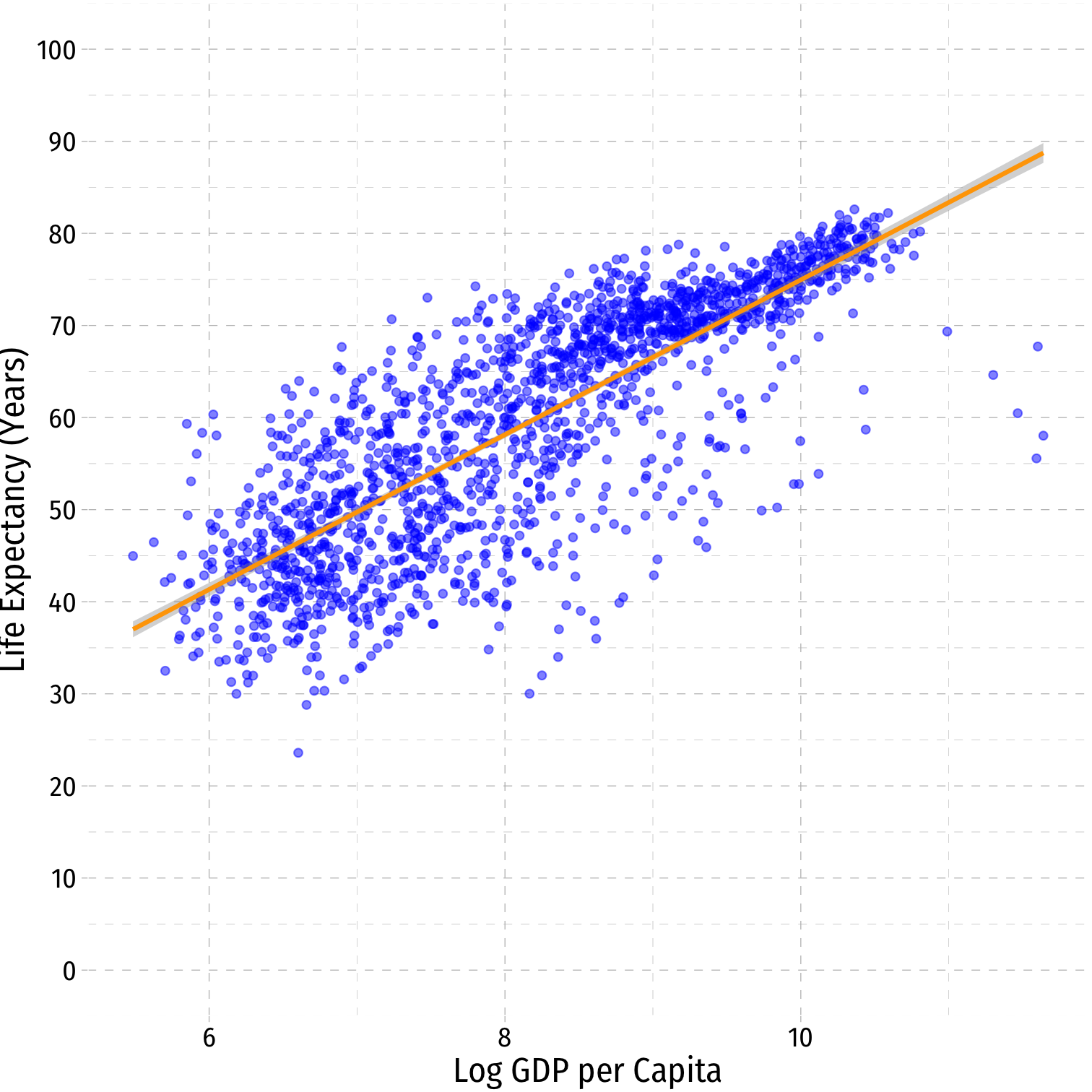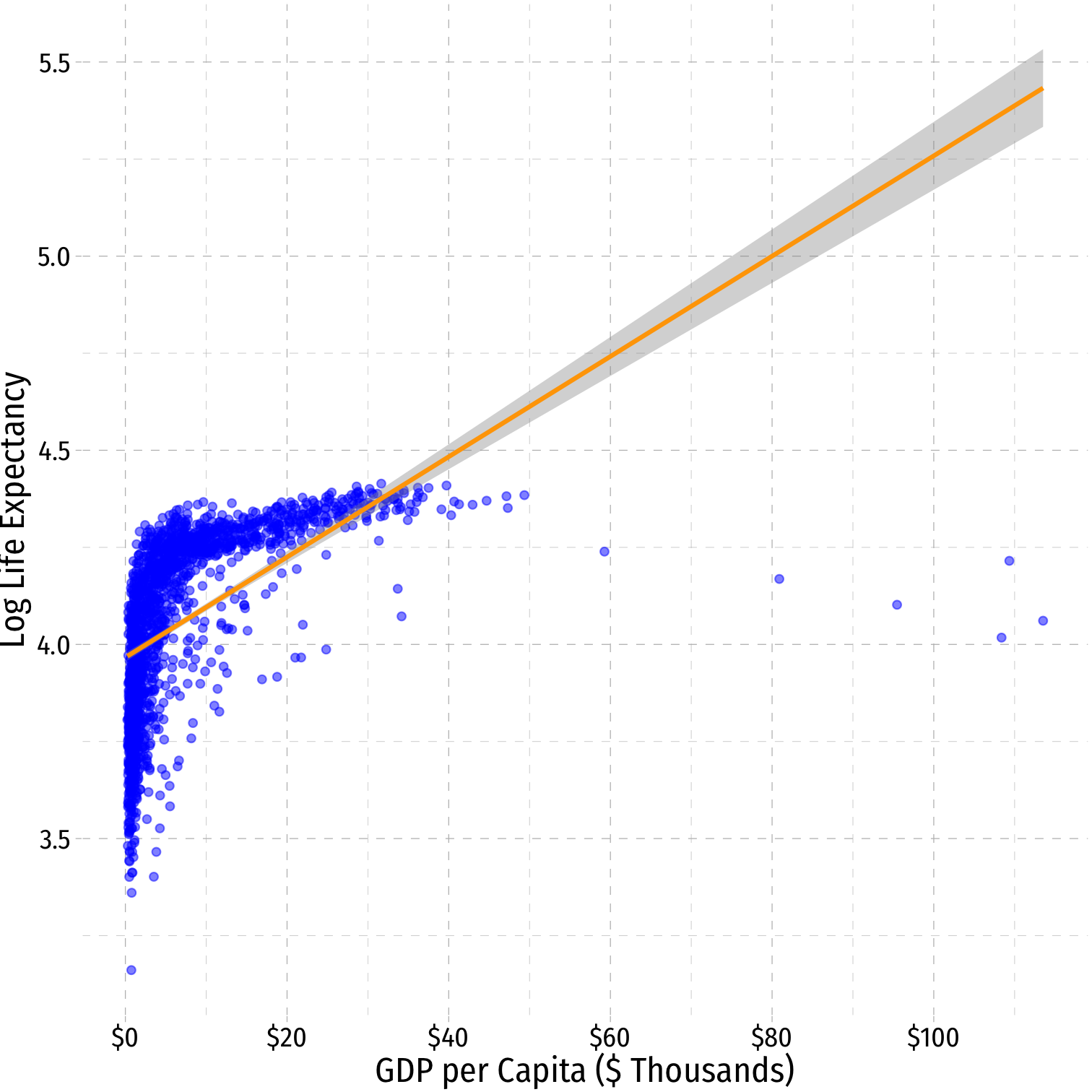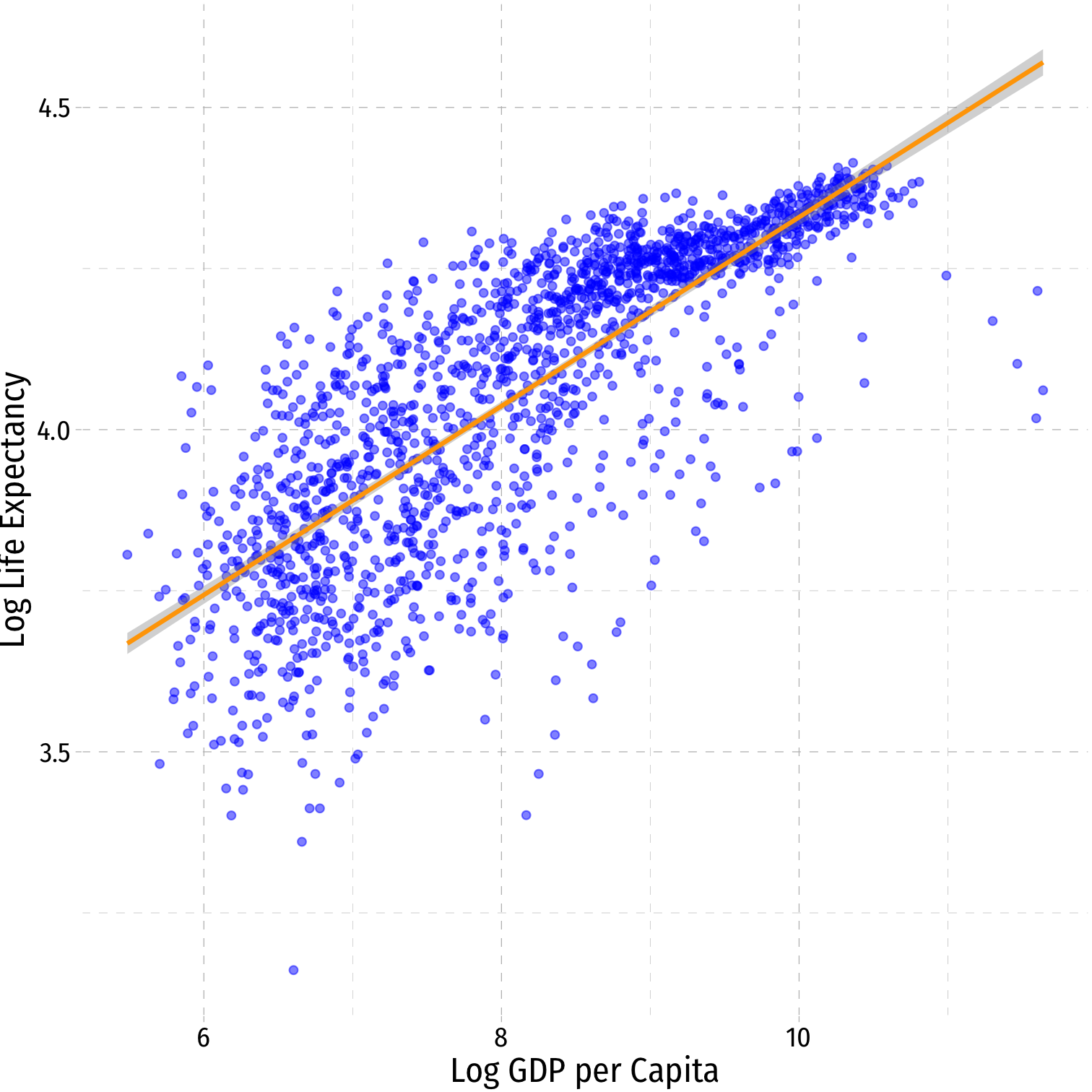Meeting Dates

Monday, November 14, 2022 Wednesday, November 16, 2022

Upcoming Assignment

Problem Set 5 is due on Monday November 28.

## Overview

This week we will cover non-linear models, which we anticipated last week with an interaction effect between two continuous variables: $$X_1 \times X_2$$, where the marginal effect of $$X_1 \rightarrow Y$$ is dependent on the value of $$X_2$$.

We cover two types of nonlinear models. First, we cover polynomial models, where we include higher-order $$X$$ variables to explain a non-linear effect.

Next we will cover logarithmic models, which are more frequently used — as well as a few final tests and transformations for multivariate regression before we turn to panel data (Unit IV) next week: standardizing variables to compare effect sizes, and joint hypothesis tests.

Interpretting logged variables can often be difficult to remember, so here I reproduce the tables that describe the interpretations of the marginal effect of $$X \rightarrow Y$$, as well as some visual examples from the slides:

Model Equation Interpretation
Linear-Log $$Y=\beta_0+\beta_1 \mathbf{ln(X)}$$ 1% change in $$X \rightarrow \frac{\hat{\beta_1}}{100}$$ unit change in $$Y$$
Log-Linear $$\mathbf{ln(Y)}=\beta_0+\beta_1X$$ 1 unit change in $$X \rightarrow \hat{\beta_1}\times 100$$% change in $$Y$$
Log-Log $$\mathbf{ln(Y)}=\beta_0+\beta_1\mathbf{ln(X)}$$ 1% change in $$X \rightarrow \hat{\beta_1}$$% change in $$Y$$
• Hint: the variable that gets logged changes in percent terms, the variable not logged changes in unit terms
Linear-Log Log-Linear Log-Log$$\hat{Y_i}=\hat{\beta_0}+\hat{\beta_1}\mathbf{ln(X_i)}$$ $$\mathbf{ln(\hat{Y_i})}=\hat{\beta_0}+\hat{\beta_1}X_i$$ $$\mathbf{ln(\hat{Y_i})}=\hat{\beta_0}+\hat{\beta_1}\mathbf{ln(X_i)}$$
$$R^2=0.65$$ $$R^2=0.30$$ $$R^2=0.61$$

After we finish, we will do another set of practice problems in R., and you will be given your final graded HW 5 to work on this material.

• Ch. 7 in Bailey, Real Econometrics

## R Practice

This week you will be working on R practice problems on nonlinear data. Answers will be posted later on that page.

## Assignments

### Problem Set 5 Due Mon Nov 28

Problem Set 5 is due by the end of the day on Wednesday, November 28.

## Slides

Below, you can find the slides in two formats. Clicking the image will bring you to the html version of the slides in a new tab. The lower button will allow you to download a PDF version of the slides.

I suggest printing the slides beforehand and using them to take additional notes in class (not everything is in the slides)!